# KS1 Maths Quiz - Year 2 Money - Recognising Pounds and Pence (Questions)

This quiz addresses the requirements of the National Curriculum KS1 Maths and Numeracy for children aged 6 and 7 in year 2. Specifically this quiz is aimed at the section dealing with recognising and using symbols for pounds (£) and pence (p) and combining amounts to make a particular value.

Children in Year 2 should be familiar with and able to recognise all the coins we use and their values. They should be able to look at a selection of coins and work out the total, perhaps by starting with the largest value coin and adding accordingly. They should also be able to recognise the symbols for pounds and pence.

This quiz will help to familiarise your child with the coins we use, their values and the symbols used for pounds and pence.

To see a larger image, click on the picture.
1. How much is here?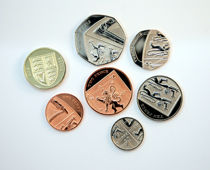[ ] £1.08 [ ] £1.88 [ ] £2.28 [ ] £1.58
2. How much is here?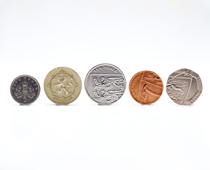[ ] £1.07 [ ] £1.60 [ ] £2.06 [ ] £1.36
3. What is the total of these coins?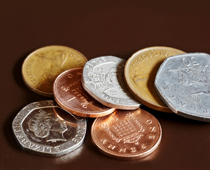[ ] 87p [ ] 95p [ ] 78p [ ] 59p
4. What is the total value of these coins?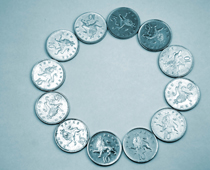[ ] £1.02 [ ] £120 [ ] £12 [ ] £1.20
5. How much money do I have here?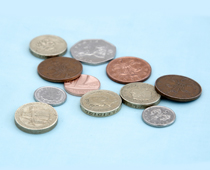[ ] £4.66 [ ] £4.76 [ ] £4.67 [ ] £4.87
6. If I added a £2 coin to this amount, how much would I have?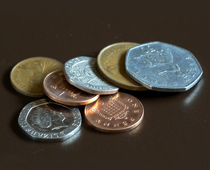[ ] £2.95 [ ] £2.55 [ ] £2.75 [ ] £2.05
7. Is this more or less than £5?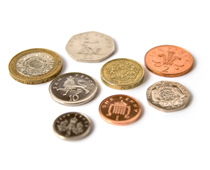[ ] Less - it's £2.25 [ ] Less - it's £3.88 [ ] More - it's £5.65 [ ] More - it's £6.88
8. How could I buy 3 potatoes using 4 coins?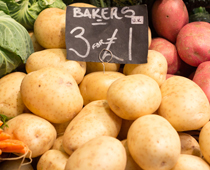[ ] 50p, 5p, 5p and 10p [ ] 50p, 10p, 10p, and 10p [ ] 20p, 20p, 20p and 20p [ ] 50p, 20p, 20p and 10p
9. If I took away all the £1 coins, how much would be left?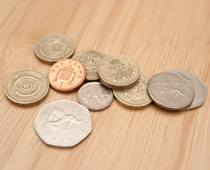[ ] £2.61 [ ] £6.16 [ ] £1.16 [ ] £1.61
10. What would be the fewest coins I could use to pay for a bunch of carrots?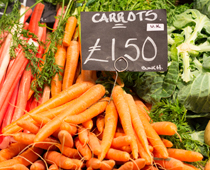[ ] 3 coins - 50p, 50p and 50p [ ] 4 coins - £1, 20p, 20p and 10p [ ] 5 coins - £1, 20p, 20p, 5p and 5p [ ] 2 coins - £1 and 50pKS1 Maths Quiz - Year 2 Money - Recognising Pounds and Pence (Answers)
1. How much is here?[ ] £1.08 [x] £1.88 [ ] £2.28 [ ] £1.58
Starting with the coin of highest value is a good addition strategy
2. How much is here?[ ] £1.07 [ ] £1.60 [ ] £2.06 [x] £1.36
Adding the smaller value coins up and then including the £1 could help
3. What is the total of these coins?[ ] 87p [x] 95p [ ] 78p [ ] 59p
Starting with 50p and counting up in 10s could be a good strategy
4. What is the total value of these coins?[ ] £1.02 [ ] £120 [ ] £12 [x] £1.20
There are twelve 10p pieces - ten of them would make £1, with two more making 20p
5. How much money do I have here?[ ] £4.66 [ ] £4.76 [x] £4.67 [ ] £4.87
Once you have found the total of the smaller coins, add the £4 on
6. If I added a £2 coin to this amount, how much would I have?[x] £2.95 [ ] £2.55 [ ] £2.75 [ ] £2.05
There is 95p here already. Adding £2.00 would give a total of £2.95
7. Is this more or less than £5?[ ] Less - it's £2.25 [x] Less - it's £3.88 [ ] More - it's £5.65 [ ] More - it's £6.88
These are all of the 8 different coins we use today
8. How could I buy 3 potatoes using 4 coins?[ ] 50p, 5p, 5p and 10p [ ] 50p, 10p, 10p, and 10p [ ] 20p, 20p, 20p and 20p [x] 50p, 20p, 20p and 10p
There are lots and lots of ways to make £1 using different coins!
9. If I took away all the £1 coins, how much would be left?[ ] £2.61 [ ] £6.16 [x] £1.16 [ ] £1.61
There is still over £1, thanks to the two 50p coins
10. What would be the fewest coins I could use to pay for a bunch of carrots?[ ] 3 coins - 50p, 50p and 50p [ ] 4 coins - £1, 20p, 20p and 10p [ ] 5 coins - £1, 20p, 20p, 5p and 5p [x] 2 coins - £1 and 50p
You could also pay with a £2 coin and get 50p change!